## conference papersJOURNAL OFAPPLIEDCRYSTALLOGRAPHY
ISSN: 1600-5767
Volume 40| Part s1| April 2007| Pages s165-s169

## Charge interaction and temperature effects on the solution structure of lysozyme as revealed by small-angle X-ray scattering

aNational Synchrotron Radiation Research Center, 101 Hsin-Ann Road, Hsinchu Science Park, Hsinchu 30076, Taiwan, and bInstitute of Atomic and Molecular Sciences, Academia Sinica, PO Box 23-166, 106 Taipei, Taiwan

(Received 16 August 2006; accepted 15 January 2007; online 10 February 2007)

We have studied the structure of lysozyme as influenced by solution environment using small-angle X-ray scattering (SAXS). With an ellipsoid form factor and a structure factor derived using the mean spherical approximation to account for the electrostatic repulsion of lysozyme, we have extracted detailed structural information about the protein in aqueous solutions, including the size, shape, and net charge number. The SAXS data analysis shows that lysozyme in pure water, expressing an averaged net charge number of ~6, folds to an ellipsoid-like shape with a radius of gyration Rg = 16.6 Å. Temperature-dependent SAXS for lysozyme in a buffer solution in which charge repulsion has been eliminated suggests that the protein may thermally unfold gradually along a preferred direction from the ellipsoidal shape with an aspect ratio of p ≃ 2 at 303 K to an elongated shape with p ≃ 3 at 343 K. The structural parameters of the unfolded lysozyme obtained using model fitting are compared with the envelope morphology simulated using a dummy-residues model. From the evolution of the volume of lysozyme during the thermal unfolding process, we deduce a free-energy profile for the protein thermally unfolded in water using a modified Ising model on the basis of a mean field approximation.

### 1. Introduction

Many aspects of the solution structure of lysozyme have been well studied, including morphology and hydration (Svergun et al., 1998; Chacon et al., 1998), folding–unfolding behavior (Chen et al., 1996; Arai & Hirai, 1999; Baldwin, 2002), clustering behavior (Stradner, 2004; Liu et al., 2005), binding with membranes (Zschornig et al., 2005), and many others. Despite being well documented in the literature, lysozyme-related research remains active, partly due to advances in tools for studies of solution structure (Fujisawa et al., 2000), including small-angle X-ray scattering (SAXS), X-ray and/or light absorption/fluorescence, and circular dichroism (CD). For proteins unfolded by various denaturants or elevated temperatures, many studies have demonstrated that SAXS can be an effective tool to probe the global structure and the structure evolution even on a sub-millisecond scale (Chen et al., 1996; Segel et al., 1999; Doniach, 2001; Uzawa et al., 2004). Of the many SAXS studies on proteins structures, the majority seems to focus on the global size and the change in size of proteins in different solution conditions, with the local chain behavior discussed qualitatively using a Kratky–Porod plot and/or the pair distribution function p(r) (Cinelli et al., 2001). The size of the protein studied is often referred to the radius of gyration Rg extracted from the corresponding SAXS data in the low-angle scattering region using the Guinier approximation. The value of Rg can certainly be used to indicate the size of a protein; however, when the unfolded protein is far from a globular shape, the Rg value may not accurately reflect the volume of the protein, due to the distance bias factor r2 of Rg. Since the volume of a protein may be used to characterize the protein unfolding behavior by using the newly developed Ising model with a mean field approximation (Liang et al., 2003), we employed model simulation (Liu et al., 2005; Tuinier & Brulet, 2003) to directly extract the shape and volume occupied by lysozyme (including the water molecules of hydration) in aqueous solutions from the measured SAXS data.

To examine the validity of the uniform density assumption used in our model fitting for the SAXS data collected for the thermally unfolded lysozyme, we compare the model-fitting result with the envelope shape of the unfolded lysozyme obtained using a simulation based on a dummy-residues model (Svergun et al., 1998). In addition, from the volume change of lysozyme during the unfolding process (extracted from the SAXS data) we can deduce a free-energy profile for the lysozyme unfolded in water, using the modified Ising model with a mean field approximation recently developed for protein folding–unfolding behavior (Liang et al., 2003).

### 2. SAXS model

Small-angle scattering profiles for monodisperse colloidal particles can be modeled as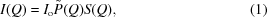where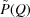is the normalized form factor with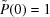, and S(Q) is the structure factor with S(Q) ≃ 1 at large Q values. Here, Q = (4π/λ)sin θ is defined by the scattering angle 2θ and the wavelength λ. The zero-angle scattering intensity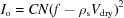is determined by the concentration C, the aggregation number N, the dry volume Vdry, the scattering length f of the scattering particles, and the scattering-length density ρs of the solvent (Chen & Lin, 1987). For lysozyme, with 7648 electrons, f = 0.21567 Å, whereas ρs = 9.43 × 10−6 Å−2 for water.

For homogeneous ellipsoids with semi-major axis a and semi-minor axis b, the form factor averaged for spatial orientation is given by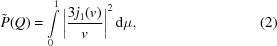where v = Q[a2μ2 + b2(1 − μ2)]1/2 and j1 is the spherical Bessel function of the first order (Feigin & Svergun, 1987).

For macro-ion solutions with non-trivial interparticle interactions, the measured SAXS profile I(Q) is a convolution of the form factor P(Q) of the scattering particles and the structure factor characterized by the electrostatic repulsion of the charged particles. To extract the particle morphology P(Q) from such a SAXS profile, one needs to account for the scattering contribution by S(Q) as well. In this study, we adapted the S(Q) values obtained from the commonly used mean spherical approximation (MSA) first developed by Hayter et al. (Hayter & Penfold, 1981). The MSA assumes rigid charged spheres of effective diameter σ and charge number Z, interacting with each other through a screened Coulomb potential. For non-spherical particles, the S(Q) values calculated from the MSA can be corrected for a non-spherical particle effect. The effective sphere diameter σ can be obtained using an equivalent volume approximation V = 4/3π(σ/2)3, where V is the volume of the particle contributing to the volume fraction η = CV/N (Lin et al., 2004). For proteins in solution with no aggregation, N = 1, and V is equivalent to the volume occupied by a single protein molecule with its water molecules of hydration. When the charge interaction between the particles is largely eliminated by salt added to the solution, the structure factor S(Q) under the MSA reduces to that derived from the Percus–Yevick model of hard-sphere interactions and may be neglected [S(Q) ≃ 1)] in solutions of low protein concentrations, i.e., low volume fractions (Chen & Lin, 1987).

### 3. Experimental details

Hen egg-white lysozyme (HEWL), purchased from Sigma Chemical Co., was used without further purification. Sample solutions containing 7, 10.5, 21, and 42 mg ml−1 lysozyme were prepared with deionized water. For protein-unfolding SAXS measurements, 100 mM NaCl and 100 mM sodium citrate buffer at pH 2.9 were added to aqueous 7, 14, and 21 mg ml−1 lysozyme solutions.

SAXS measurements were conducted on the SAXS instrument of the BL01B beamline at the National Synchrotron Radiation Research Center (NSRRC). Details of the SAXS instrumentation have been reported previously (Lai et al., 2005). For better scattering intensity, a 1 mm diameter beam of 15 keV (0.827 Å) high-energy photons and an optimum sample thickness of ~7 mm were used (for a transmission factor of ~0.37), with a sample-to-detector distance of 1.42 m. During the SAXS data collection with a 50 mm long linear detector of 80 µm pixel resolution, samples sealed by 12 µm thick Kapton windows (5 mm diameter) were oscillated slowly over an area of 2 × 2 mm to prevent long irradiation in one place, which proved to be effective in preventing aggregation during the typical data-aquisition time of 30 min. Using the position sensitive gas proportional linear detector, we could monitor in real time the SAXS profiles as they steadily built up. The time-independent SAXS profiles observed during the data accumulation showed no formation of a hump or a rising peak in the low-Q region, implying little aggregation effect.

### 4. Results and discussion

#### 4.1. Interparticle interactions

All four sets of SAXS data measured for the sample solutions of 7, 10.5, 21, and 42 mg ml−1 lysozyme in pure water (shown in Fig. 1) demonstrate a prominent interaction hump, the peak position of which shifts to a higher Q value as the concentration increases. Considering the low lysozyme volume fractions in these solutions (a few per cent at most), of a small excluding volume effect, we attribute the significant interaction peak mainly to the electrostatic interaction between the lysozyme molecules in water. To extract detailed structural information, we fitted the SAXS data with an ellipsoid form factor and a structure factor from the MSA model as described previously. To reduce the fitting parameters, we used the absolute intensity in our data fitting and fitted the four sets of SAXS data simultaneously with the four common parameters of (1) the semi-major axis a and (2) the semi-minor axis b of the ellipsoid, (3) the dry volume Vdry, and (4) the net charge number Z of lysozyme (Lin et al., 2004). In the fitting algorithm, we have taken into account the smearing effect of Q due to the instrument resolution, and we used an aggregation number N = 1 for the well dispersed protein solutions together with an effective hard-sphere diameter σ = 2(ab2)1/3 for the MSA model based on an equivalent volume approximation. As a result, the four sets of data can be described adequately (see the corresponding simulations in Fig. 1) using the four common parameters fitted: a = 31.3 ± 2.3 Å, b = 14.1 ± 0.5 Å, Vdry = 17840 ± 120 Å3, and Z = 5.7 ± 0.3.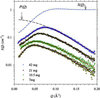Figure 1 The SAXS profiles of 7, 10.5, 21, and 42 mg ml−1 aqueous lysozyme solutions are fitted (dashed curves) with the same normalized ellipsoid form factortogether with the respective structure factors S(Q). Also shown are the P(Q) and S(Q) curves for the sample solution of 42 mg ml−1 lysozyme.

The dry volume obtained is consistent with the specific volume of 17 960 Å3 for lysozyme previously reported from density measurement (Orthaber et al., 2000; Narayanan & Liu, 2003). We emphasize the necessity of using SAXS data on an absolute intensity scale in the fitting algorithm to obtain the dry volume of the protein, the value of which relates closely to the absolute intensity of the zero-angle scattering. Furthermore, the charge number from the fit is consistent with the value of 6.8 ± 1.5 given by Liu et al. for a buffer solution with a high lysozyme concentration of 20% (Liu et al., 2005). The simultaneous fit of the four sets of SAXS data here apparently gives a stronger constraint, thus a smaller uncertainty for the net charge number. On the other hand, using the two axes of the ellipsoid fitted using the equation Rg = [(a2 + 2b2/5)]1/2 (Feigin & Svergun, 1987), we deduce a value of Rg = 16.6 ± 1.0 Å. From the dry volume of lysozyme, we can deduce a hydration number NH = 270 for lysozyme using NH = (VellipsoidVd)/Vw, with Vw = 30.3 Å3 for the volume of a water molecule (Jeng et al., 2002), assuming the ellipsoid fitted is composed of a lysozyme molecule with water of hydration.

The net charge number of 6 for the protein in pure water (pH ≃ 7) that was extracted from the SAXS data analysis may be contributed by the amino acids near the surface of the protein. After detailed examination of the protein structure based on files from the Protein Data Bank (PDB), we suggest that the net charge may be the collective effect of the 18 charged amino acids located near the protein surface, including six negatively charged and twelve positively charged amino acids: Glu7(−), Arg14(+), His15(+), Lys96(+), Lys97(+), and Asp101(−) in the α helix; Arg45(+) in the β sheet; and Lys1(+), Asp18(−), Arg21(+), Asp48(−), Arg73(+), Asp87(−), Arg114(+), Lys116(+), Asp119(−), Arg125(+), and Arg128(+) in the loops. The loops of lysozyme are rich in charges and degree of freedoms, and may dominate the charge interaction behavior of the protein.

We also measured SAXS from the sample solutions with 7, 14, and 21 mg ml−1 lysozyme, each containing 100 mM NaCl and 100 mM sodium citrate buffer at pH 2.9. With salt added to the buffer solutions, the interparticle interaction due to the electrostatic repulsion is largely eliminated, as can be seen from the good overlap of the SAXS profiles (normalized to the concentration) in the inset of Fig. 2. From the three sets of SAXS data, we can extract a common Rg value of 15.7 ± 0.1 Å using the Guinier approximation. Furthermore, when the salt concentration in the sample solution of 21 mg ml−1 lysozyme is gradually reduced from 0.1 M NaCl to 0.03 M and then to zero, the SAXS profile changes slightly, indicating the existence of residual interparticle interaction. In Fig. 2, we show that all three sets of SAXS data for the sample solutions containing 0, 0.03, and 0.1 M NaCl can be fitted with the Guinier approximation with Rg values of 15.0, 15.2, and 15.7 ± 0.1 Å, respectively. The result implies that overlooking the residual interparticle interaction effect may lead to an underestimated Rg value.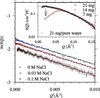Figure 2 Guinier approximations (dashed lines) with Rg values of 15.0, 15.2, and 15.7 Å for the SAXS data from 21 mg ml−1 lysozyme in a buffer solution at pH 2.9, with 0, 0.03, and 0.1 M NaCl added. The inset shows the good overlap of the SAXS data (normalized to concentration C) for the three sample solutions with 21, 14, and 7 mg ml−1 lysozyme in the same buffer solution with 0.1 M NaCl. These sets of data are fitted (dashed curve) with the same ellipsoid form factor. For comparison, SAXS data for 21 mg ml−1 lysozyme in pure water show a prominent interference peak in the low-Q region.

#### 4.2. Thermal unfolding

Temperature-induced unfolding of lysozyme has been reported previously (Arai & Hirai, 1999), where the concentration effect on the Rg value extracted using the Guinier approximation was mentioned. In that report, the Rg value was derived from the pair correlation function Fourier transformed from the SAXS data. Here, we use the same model simulation mentioned previously, with the screening effect of salt and buffer ion pairs taken into account in the MSA model (Jeng et al., 2002). We intended to directly extract size and shape information for lysozyme unfolded at elevated temperatures in a buffer solution with salt added, where the electrostatic repulsion was eliminated largely as demonstrated in the previous section.

Fig. 3shows the SAXS data collected for the 21 mg ml−1 lysozyme solution containing 100 mM NaCl and 100 mM sodium citrate buffer at pH 2.9. The temperature-dependent SAXS data were collected in the range of 303 to 343 K. The SAXS profile changes drastically at T = 343 K, indicating that the protein is largely unfolded. Using the same ellipsoid form factor and the structure factor from the MSA model with the salt concentration of the solution taken into account, we can fit the temperature-dependent SAXS data reasonably, as shown in Fig. 3. The structural parameters from the fit are summarized in Table 1. The Rg values deduced from the fitted values for the semi-major and semi-minor axes of the ellipsoid are indeed slightly larger that obtained using the Guinier approximation (see Table 1) for the same sets of data. In the inset to Fig. 3, the aspect ratio p (= a/b) of the ellipsoidal shape fitted for the thermally unfolded lysozyme grows from p ≃ 2 at 303 K to p ≃ 3 at 343 K, while the semi-minor axis b stays roughly the same length (see also Table 1). This result indicates that lysozyme may unfold in a preferred direction in the solution.

 Table 1Structural parameters extracted from the temperature-dependent SAXS data collected for a 21 mg ml−1 lysozyme solution in a buffer solution at different temperatures
 a and b are the semi-major and semi-minor axes of the ellipsoid form factor. The Rg values are obtained from a and b. Rg,GA denotes the radius of gyration obtained from the Guinier approximation.
Temperature (K) a (Å) b (Å) Rg (Å) Rg,GA (Å)
303 31.7 15.7 17.3 15.7
313 31.3 14.6 16.8 15.1
323 34.0 14.5 17.8 15.2
333 37.4 14.1 20.4 16.4
343 56.9 17.0 27.6 23.4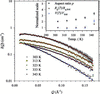Figure 3 Temperature-dependent SAXS data collected for a lysozyme solution containing buffer and salt. The data are fitted (dashed curves) with an ellipsoid form factor together with the MSA structure factor. The inset shows the aspect ratio p of the ellipsoid form factor fitted for each set of SAXS data. Also shown are the volume and Rg values extracted from the ellipsoid shapes, with these two values normalized to that measured at 303 K.

Fig. 4shows the p(r) functions Fourier transformed from the SAXS data measured at 303, 333, and 343 K. The p(r) function thus obtained is fitted using a dummy-residues simulation (Svergun et al., 1998; Koch et al., 2003) using the corresponding lysozyme morphology shown with the hydration water shell effect taken into account. Overall, the simulated molecular envelope of the folded lysozyme at 303 K is consistent with the ellipsoid shape obtained with the previous model calculation. Furthermore, the envelope obtained for lysozyme at 343 K also consistently displays a stretched shape with an aspect ratio ~3, as revealed by the SAXS model fitting with ellipsoids. Nevertheless, we found that the Rg values (15.5 Å at 303 K and 24.5 Å at 343 K) of the protein extracted from the p(r) function by the program GNOM are somewhat lower than that obtained using the ellipsoid model calculation (Table 1). It is likely that the p(r) function may be influenced by hard-sphere interactions of lysozyme.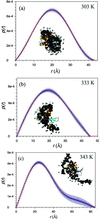Figure 4 The pair correlation functions p(r) (circles) Fourier-transformed (using GNOM) from the SAXS data collected at (a) 303, (b) 333 and (c) 343 K, are fitted (solid curves) with dummy-residues modelling (using the program GASBOR) with the corresponding lysozyme envelopes shown by the black spheres. Also shown are water molecules (light spheres) surrounding the lysozyme and the crystal structure of lysozyme (ribbons).

#### 4.3. Protein unfolding model and the free-energy parameters

In the Ising model with a mean field approximation developed recently for protein unfolding (Liang et al., 2003), a protein is regarded as an ensemble of units (averaged over the couplings among the units) with each unit being in either the folded or unfolded state. The unfolding fraction ratio, governed by the free energy of the system, is expressed as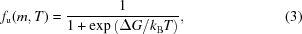with the free energy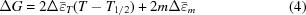depending on the temperature T and the denaturant concentration m, when the couplings among the units can be ignored. The denature energy parameters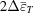and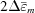associated with the temperature effect and the denaturant concentration effect can, respectively, be extracted from the measurements for proteins denatured by temperature or denaturant. Furthermore, without denaturant, i.e. m = 0, the free energy of native proteins dissolved in water can be reduced to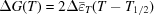, where T1/2 represents the temperature of the middle point of the unfolding fraction ratio (fu = 0.5). Thus,in this specific case can be related to the entropy effect in the general relation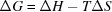, according to equation (4).

Fig. 5shows a plot of the unfolding fraction ratio of lysozyme in terms of the volume ratio extracted from the SAXS data (inset of Fig. 3). We have taken the most concise volume measured at 303 K as the folded state of lysozyme (corresponding to fu = 0) and the largest volume measured at 343 K as the unfolded state (corresponding to fu = 1). Fitting the unfolding fraction ratio using equation (4)(the solid curve in Fig. 5), we can deduce that= −2.971 kJ mol−1 K−1, with T1/2 = 335 K, for the 21 mg ml−1 lysozyme solution containing buffer at pH 2.9. In the inset to Fig. 5, we show that the temperature-dependent free energies calculated using equation (4)using this value offollow the general thermodynamic relationship, indicating thatcan be associated with ΔS in this case. The free energy of lysozyme at 303 K obtained here, ΔG(303 K) ≃ 105 kJ mol−1, is much larger than the value of 61.5 kJ mol−1 obtained in a similar way for cytochrome c, implying that lysozyme may have a higher stability in the same solution than cytochrome c does. This result is likely to be related to the more compact structure of native lysozyme (in which more amino acids form the secondary structure) than cytochrome c. We note that the ΔG value obtained here for lysozyme may suffer a large uncertainty (few tens of per cents) due to the limited number data points available.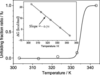Figure 5 Temperature-dependent unfolding fraction ratio of lysozyme in a buffer solution of pH = 2.9. The inset show the entropy ΔS = 0.71 kcal mol−1 K−1 extracted from the slope of the solid fitted line using ΔG = ΔH − TΔS.

### 5. Summary and conclusions

We have studied the charge interaction and temperature effects on the solution structure of lysozyme with SAXS. Using an ellipsoid form factor and the MSA structure factor for SAXS data analysis, we have deduced the size, shape, and net charge number of lysozyme. The charge number can be correlated with the charged amino acids on the protein surface. From temperature-dependent SAXS, lysozyme seems to unfold along a preferred direction, and the aspect ratio of the ellipsoid-like protein grows from 2 in the folded state to 3 in the unfolded state. We have demonstrated that the free energy of the protein for thermal unfolding can also be extracted from the temperature-dependent SAXS data using the Ising model with a mean field approximation. Dummy-residues simulation was employed to provide another view on the solution morphology of lysozyme, especially that of the unfolded lysozyme.

### Acknowledgements

We appreciate the assistance of Drs T. Fujusawa and M. Petoukhov in the data fitting using the programs CRYSOL, GNOM, and GASBOR. The work was partially supported by the NSC under grant No. NSC-95-2112-M-213-002.

### ReferencesJOURNAL OFAPPLIEDCRYSTALLOGRAPHY